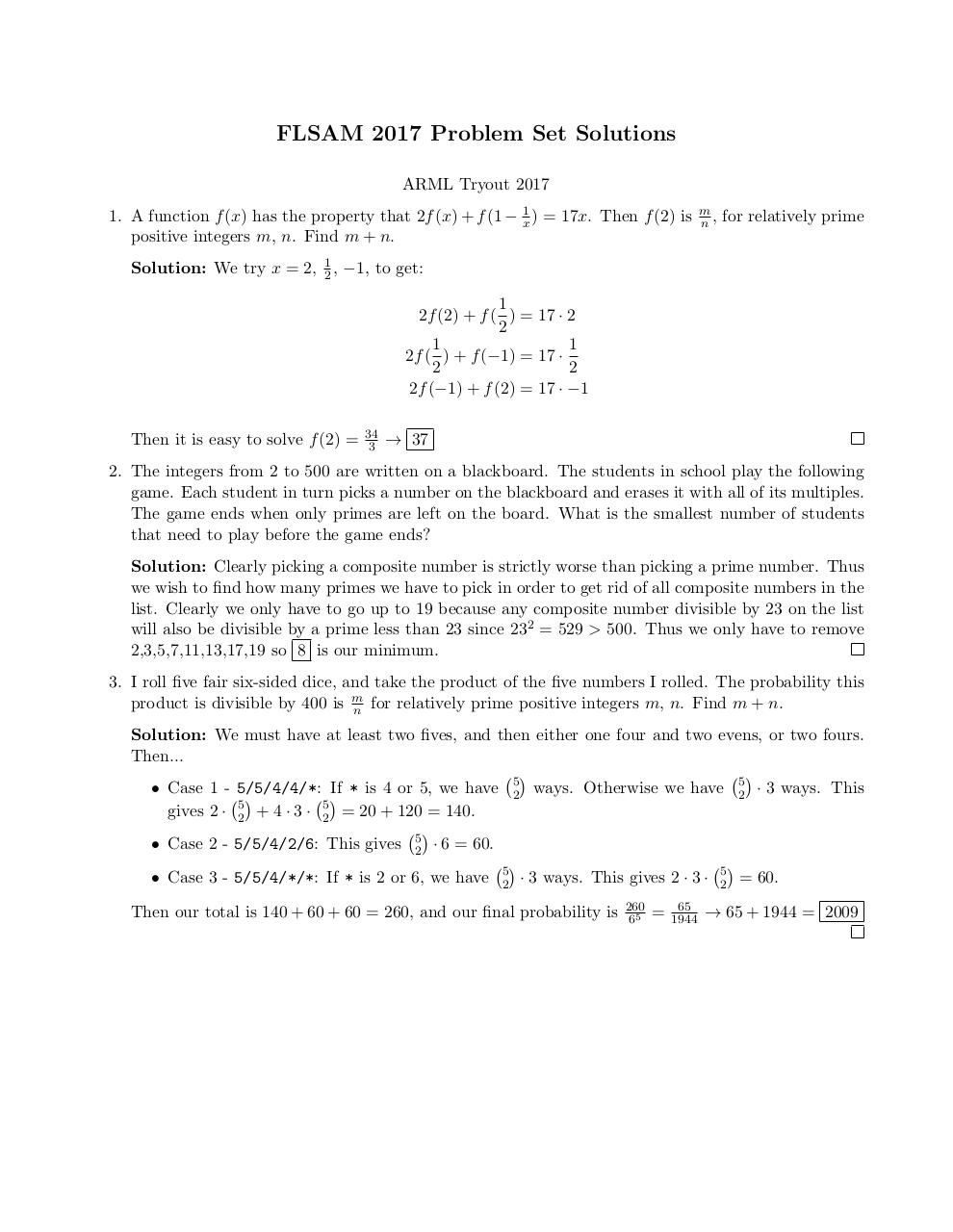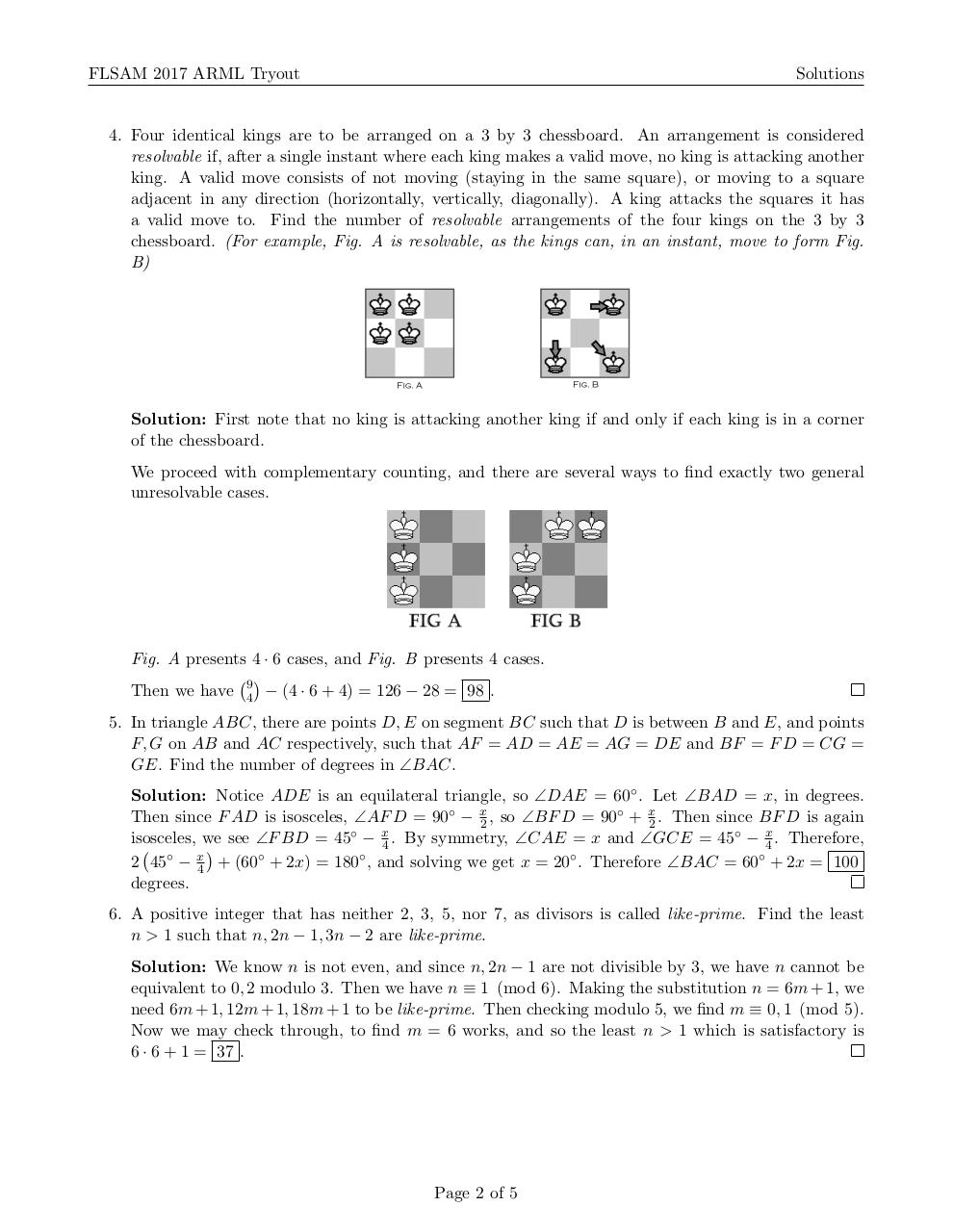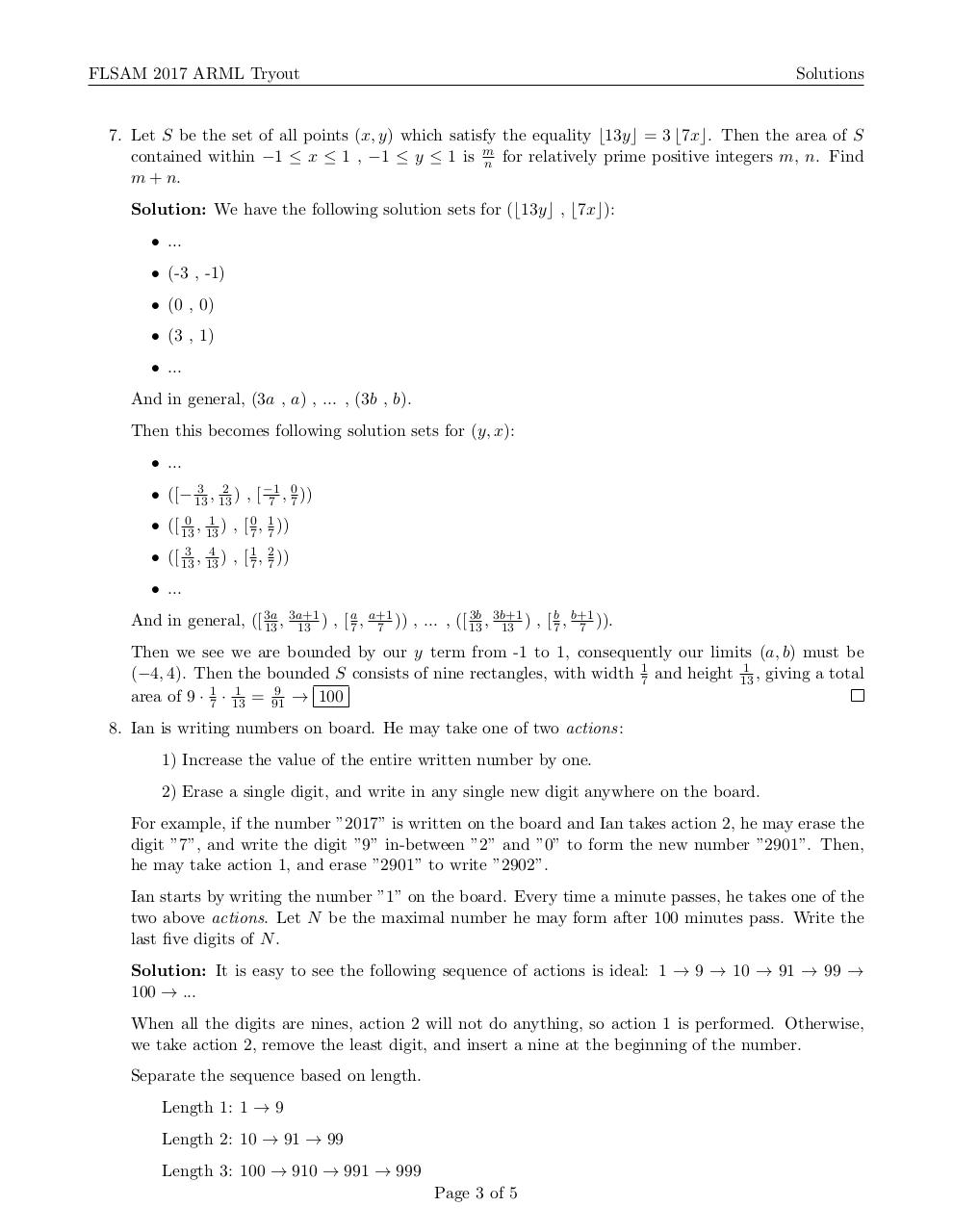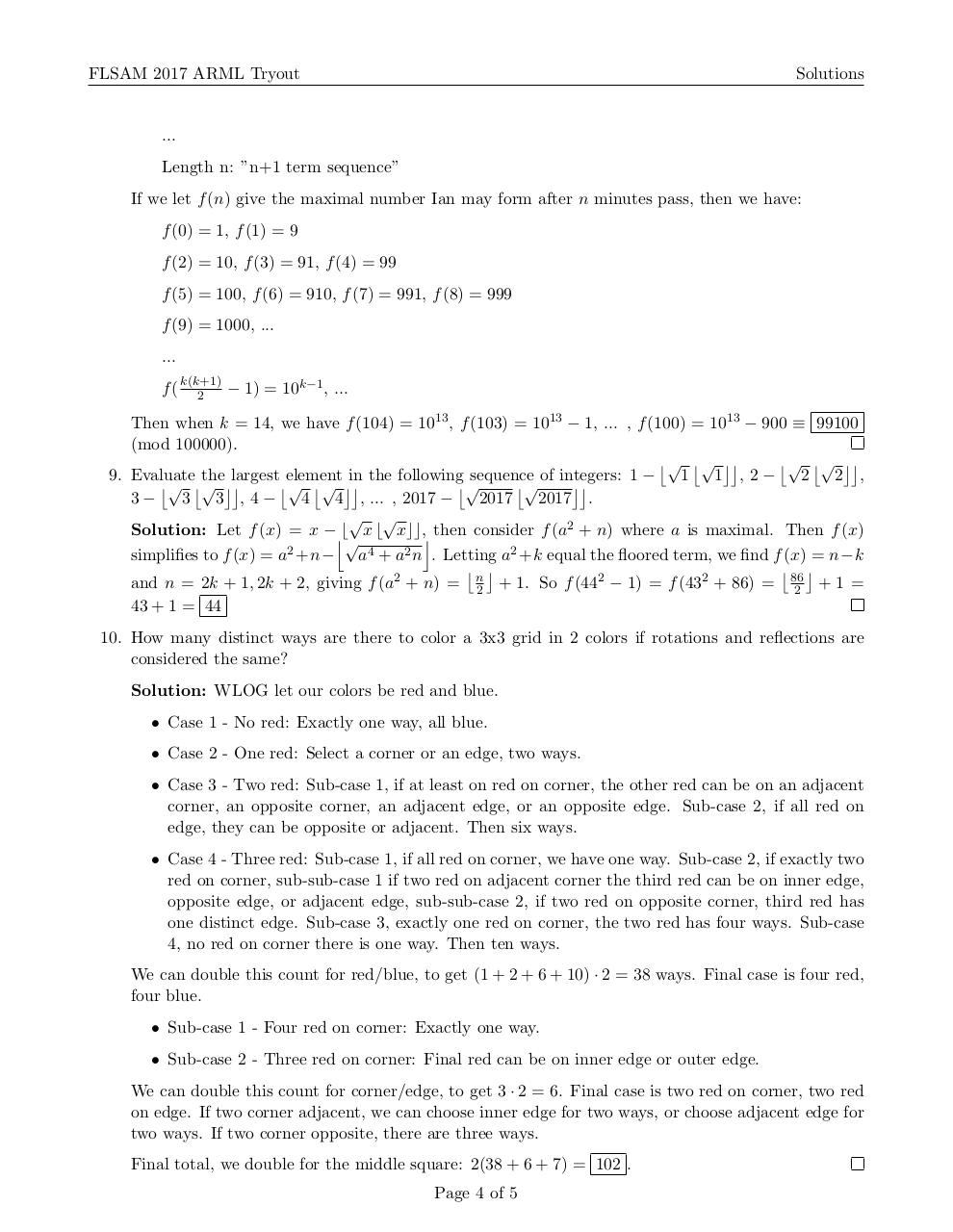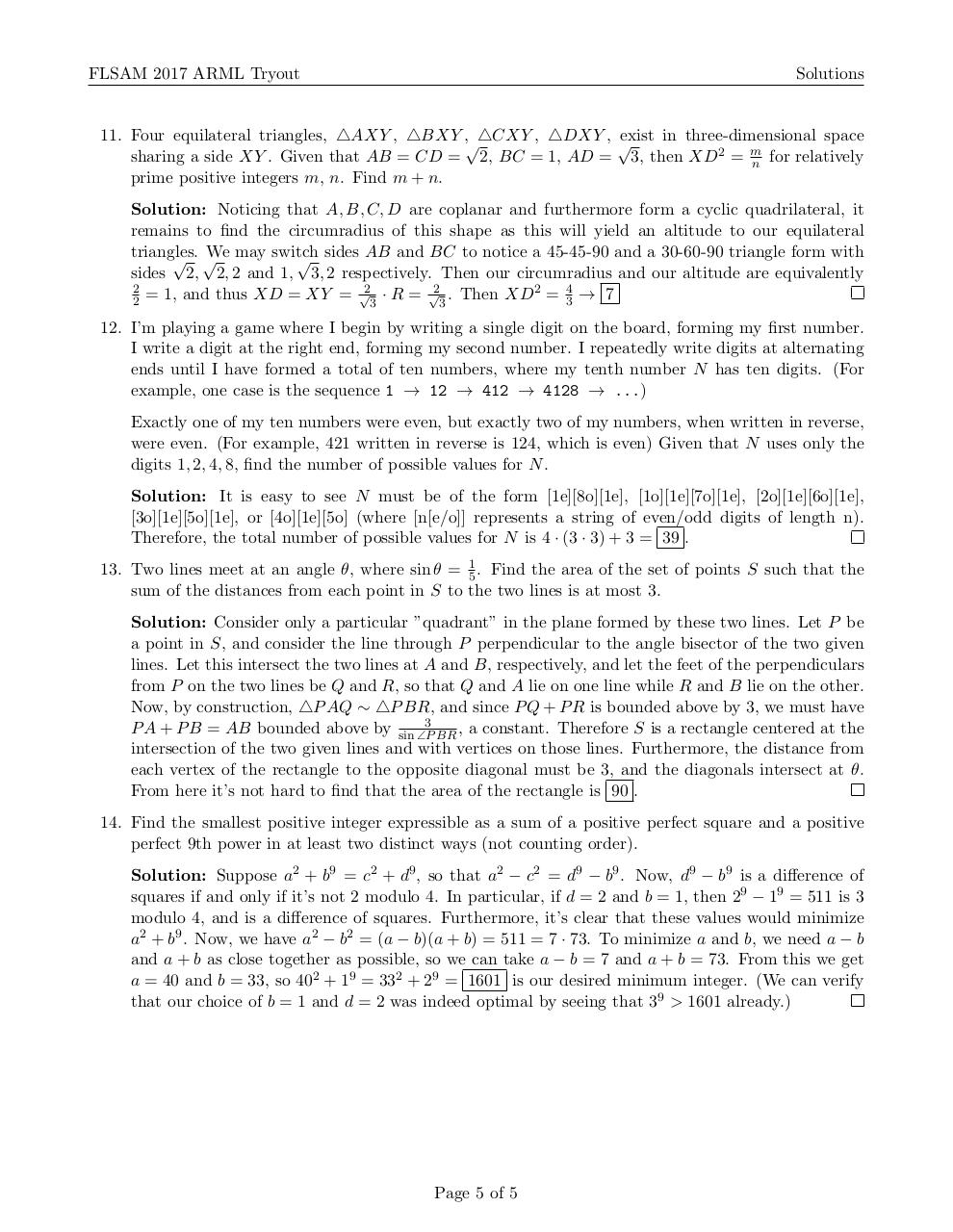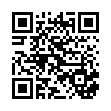# FLSAM 2017 ARML Tryout Solutions compact .pdf

### File information

Original filename: FLSAM_2017_ARML_Tryout__Solutions_compact_.pdf

This PDF 1.5 document has been generated by TeX / pdfTeX-1.40.17, and has been sent on pdf-archive.com on 15/04/2017 at 22:52, from IP address 107.77.x.x. The current document download page has been viewed 530 times.
File size: 187 KB (5 pages).
Privacy: public file

FLSAM_2017_ARML_Tryout__Solutions_compact_.pdf (PDF, 187 KB)

### Document preview

FLSAM 2017 Problem Set Solutions
ARML Tryout 2017
1. A function f (x) has the property that 2f (x) + f (1 − x1 ) = 17x. Then f (2) is
positive integers m, n. Find m + n.

m
n,

for relatively prime

Solution: We try x = 2, 12 , −1, to get:
1
2f (2) + f ( ) = 17 · 2
2
1
1
2f ( ) + f (−1) = 17 ·
2
2
2f (−1) + f (2) = 17 · −1
Then it is easy to solve f (2) =

34
3

→ 37

2. The integers from 2 to 500 are written on a blackboard. The students in school play the following
game. Each student in turn picks a number on the blackboard and erases it with all of its multiples.
The game ends when only primes are left on the board. What is the smallest number of students
that need to play before the game ends?
Solution: Clearly picking a composite number is strictly worse than picking a prime number. Thus
we wish to find how many primes we have to pick in order to get rid of all composite numbers in the
list. Clearly we only have to go up to 19 because any composite number divisible by 23 on the list
will also be divisible by a prime less than 23 since 232 = 529 &gt; 500. Thus we only have to remove
2,3,5,7,11,13,17,19 so 8 is our minimum.
3. I roll five fair six-sided dice, and take the product of the five numbers I rolled. The probability this
product is divisible by 400 is m
n for relatively prime positive integers m, n. Find m + n.
Solution: We must have at least two fives, and then either one four and two evens, or two fours.
Then...


• Case 1 - 5/5/4/4/*: If * is 4 or 5, we have 52 ways. Otherwise we have 52 · 3 ways. This


gives 2 · 52 + 4 · 3 · 52 = 20 + 120 = 140.

• Case 2 - 5/5/4/2/6: This gives 52 · 6 = 60.


• Case 3 - 5/5/4/*/*: If * is 2 or 6, we have 52 · 3 ways. This gives 2 · 3 · 52 = 60.
Then our total is 140 + 60 + 60 = 260, and our final probability is

260
65

=

65
1944

→ 65 + 1944 = 2009

FLSAM 2017 ARML Tryout

Solutions

4. Four identical kings are to be arranged on a 3 by 3 chessboard. An arrangement is considered
resolvable if, after a single instant where each king makes a valid move, no king is attacking another
king. A valid move consists of not moving (staying in the same square), or moving to a square
adjacent in any direction (horizontally, vertically, diagonally). A king attacks the squares it has
a valid move to. Find the number of resolvable arrangements of the four kings on the 3 by 3
chessboard. (For example, Fig. A is resolvable, as the kings can, in an instant, move to form Fig.
B)

Solution: First note that no king is attacking another king if and only if each king is in a corner
of the chessboard.
We proceed with complementary counting, and there are several ways to find exactly two general
unresolvable cases.

Fig. A presents 4 · 6 cases, and Fig. B presents 4 cases.

Then we have 94 − (4 · 6 + 4) = 126 − 28 = 98 .
5. In triangle ABC, there are points D, E on segment BC such that D is between B and E, and points
F, G on AB and AC respectively, such that AF = AD = AE = AG = DE and BF = F D = CG =
GE. Find the number of degrees in ∠BAC.
Solution: Notice ADE is an equilateral triangle, so ∠DAE = 60◦ . Let ∠BAD = x, in degrees.
Then since F AD is isosceles, ∠AF D = 90◦ − x2 , so ∠BF D = 90◦ + x2 . Then since BF D is again
isosceles, we see ∠F BD = 45◦ − x4 . By symmetry, ∠CAE = x and ∠GCE = 45◦ − x4 . Therefore,

2 45◦ − x4 + (60◦ + 2x) = 180◦ , and solving we get x = 20◦ . Therefore ∠BAC = 60◦ + 2x = 100
degrees.
6. A positive integer that has neither 2, 3, 5, nor 7, as divisors is called like-prime. Find the least
n &gt; 1 such that n, 2n − 1, 3n − 2 are like-prime.
Solution: We know n is not even, and since n, 2n − 1 are not divisible by 3, we have n cannot be
equivalent to 0, 2 modulo 3. Then we have n ≡ 1 (mod 6). Making the substitution n = 6m + 1, we
need 6m + 1, 12m + 1, 18m + 1 to be like-prime. Then checking modulo 5, we find m ≡ 0, 1 (mod 5).
Now we may check through, to find m = 6 works, and so the least n &gt; 1 which is satisfactory is
6 · 6 + 1 = 37 .

Page 2 of 5

FLSAM 2017 ARML Tryout

Solutions

7. Let S be the set of all points (x, y) which satisfy the equality b13yc = 3 b7xc. Then the area of S
contained within −1 ≤ x ≤ 1 , −1 ≤ y ≤ 1 is m
n for relatively prime positive integers m, n. Find
m + n.
Solution: We have the following solution sets for (b13yc , b7xc):
• ...
• (-3 , -1)
• (0 , 0)
• (3 , 1)
• ...
And in general, (3a , a) , ... , (3b , b).
Then this becomes following solution sets for (y, x):
• ...
3 2
0
• ([− 13
, 13 ) , [ −1
7 , 7 ))
0 1
, 13 ) , [ 07 , 17 ))
• ([ 13
3 4
• ([ 13
, 13 ) , [ 17 , 27 ))

• ...
3a+1
a a+1
3b 3b+1
b b+1
And in general, ([ 3a
13 , 13 ) , [ 7 , 7 )) , ... , ([ 13 , 13 ) , [ 7 , 7 )).

Then we see we are bounded by our y term from -1 to 1, consequently our limits (a, b) must be
1
(−4, 4). Then the bounded S consists of nine rectangles, with width 17 and height 13
, giving a total
1
9
area of 9 · 17 · 13
= 91
→ 100
8. Ian is writing numbers on board. He may take one of two actions:
1) Increase the value of the entire written number by one.
2) Erase a single digit, and write in any single new digit anywhere on the board.
For example, if the number ”2017” is written on the board and Ian takes action 2, he may erase the
digit ”7”, and write the digit ”9” in-between ”2” and ”0” to form the new number ”2901”. Then,
he may take action 1, and erase ”2901” to write ”2902”.
Ian starts by writing the number ”1” on the board. Every time a minute passes, he takes one of the
two above actions. Let N be the maximal number he may form after 100 minutes pass. Write the
last five digits of N .
Solution: It is easy to see the following sequence of actions is ideal: 1 → 9 → 10 → 91 → 99 →
100 → ...
When all the digits are nines, action 2 will not do anything, so action 1 is performed. Otherwise,
we take action 2, remove the least digit, and insert a nine at the beginning of the number.
Separate the sequence based on length.
Length 1: 1 → 9
Length 2: 10 → 91 → 99
Length 3: 100 → 910 → 991 → 999
Page 3 of 5

FLSAM 2017 ARML Tryout

Solutions

...
Length n: ”n+1 term sequence”
If we let f (n) give the maximal number Ian may form after n minutes pass, then we have:
f (0) = 1, f (1) = 9
f (2) = 10, f (3) = 91, f (4) = 99
f (5) = 100, f (6) = 910, f (7) = 991, f (8) = 999
f (9) = 1000, ...
...
f ( k(k+1)
− 1) = 10k−1 , ...
2
Then when k = 14, we have f (104) = 1013 , f (103) = 1013 − 1, ... , f (100) = 1013 − 900 ≡ 99100
(mod 100000).
√ √ 
√ √ 
9. Evaluate the largest element in the following sequence of integers: 1 −
1 1 , 2−
2 2 ,
√ √ 
√
√

√ √ 
3 3 , 4−
4 4 , ... , 2017 −
2017 2017 .
3−
√ √
Solution: Let f (x) = x −jb x b xcc,k then consider f (a2 + n) where a is maximal. Then f (x)

simplifies to f (x) = a2 +n− a4 + a2 n . Letting a2 +k equal the floored term, we find f (x) = n−k
 
 
and n = 2k + 1, 2k + 2, giving f (a2 + n) = n2 + 1. So f (442 − 1) = f (432 + 86) = 86
2 +1 =
43 + 1 = 44
10. How many distinct ways are there to color a 3x3 grid in 2 colors if rotations and reflections are
considered the same?
Solution: WLOG let our colors be red and blue.
• Case 1 - No red: Exactly one way, all blue.
• Case 2 - One red: Select a corner or an edge, two ways.
• Case 3 - Two red: Sub-case 1, if at least on red on corner, the other red can be on an adjacent
corner, an opposite corner, an adjacent edge, or an opposite edge. Sub-case 2, if all red on
edge, they can be opposite or adjacent. Then six ways.
• Case 4 - Three red: Sub-case 1, if all red on corner, we have one way. Sub-case 2, if exactly two
red on corner, sub-sub-case 1 if two red on adjacent corner the third red can be on inner edge,
opposite edge, or adjacent edge, sub-sub-case 2, if two red on opposite corner, third red has
one distinct edge. Sub-case 3, exactly one red on corner, the two red has four ways. Sub-case
4, no red on corner there is one way. Then ten ways.
We can double this count for red/blue, to get (1 + 2 + 6 + 10) · 2 = 38 ways. Final case is four red,
four blue.
• Sub-case 1 - Four red on corner: Exactly one way.
• Sub-case 2 - Three red on corner: Final red can be on inner edge or outer edge.
We can double this count for corner/edge, to get 3 · 2 = 6. Final case is two red on corner, two red
on edge. If two corner adjacent, we can choose inner edge for two ways, or choose adjacent edge for
two ways. If two corner opposite, there are three ways.
Final total, we double for the middle square: 2(38 + 6 + 7) = 102 .
Page 4 of 5

FLSAM 2017 ARML Tryout

Solutions

11. Four equilateral triangles, 4AXY , 4BXY ,√4CXY , 4DXY , √
exist in three-dimensional space
sharing a side XY . Given that AB = CD = 2, BC = 1, AD = 3, then XD2 = m
n for relatively
prime positive integers m, n. Find m + n.
Solution: Noticing that A, B, C, D are coplanar and furthermore form a cyclic quadrilateral, it
remains to find the circumradius of this shape as this will yield an altitude to our equilateral
triangles.
We may switch
√ √
√ sides AB and BC to notice a 45-45-90 and a 30-60-90 triangle form with
sides 2, 2, 2 and 1, 3, 2 respectively. Then our circumradius and our altitude are equivalently
4
2
2
√2
√2
2 = 1, and thus XD = XY = 3 · R = 3 . Then XD = 3 → 7
12. I’m playing a game where I begin by writing a single digit on the board, forming my first number.
I write a digit at the right end, forming my second number. I repeatedly write digits at alternating
ends until I have formed a total of ten numbers, where my tenth number N has ten digits. (For
example, one case is the sequence 1 → 12 → 412 → 4128 → ...)
Exactly one of my ten numbers were even, but exactly two of my numbers, when written in reverse,
were even. (For example, 421 written in reverse is 124, which is even) Given that N uses only the
digits 1, 2, 4, 8, find the number of possible values for N .
Solution: It is easy to see N must be of the form [1e][8o][1e], [1o][1e][7o][1e], [2o][1e][6o][1e],
[3o][1e][5o][1e], or [4o][1e][5o] (where [n[e/o]] represents a string of even/odd digits of length n).
Therefore, the total number of possible values for N is 4 · (3 · 3) + 3 = 39 .
13. Two lines meet at an angle θ, where sin θ = 15 . Find the area of the set of points S such that the
sum of the distances from each point in S to the two lines is at most 3.
Solution: Consider only a particular ”quadrant” in the plane formed by these two lines. Let P be
a point in S, and consider the line through P perpendicular to the angle bisector of the two given
lines. Let this intersect the two lines at A and B, respectively, and let the feet of the perpendiculars
from P on the two lines be Q and R, so that Q and A lie on one line while R and B lie on the other.
Now, by construction, 4P AQ ∼ 4P BR, and since P Q + P R is bounded above by 3, we must have
3
P A + P B = AB bounded above by sin ∠P
BR , a constant. Therefore S is a rectangle centered at the
intersection of the two given lines and with vertices on those lines. Furthermore, the distance from
each vertex of the rectangle to the opposite diagonal must be 3, and the diagonals intersect at θ.
From here it’s not hard to find that the area of the rectangle is 90 .
14. Find the smallest positive integer expressible as a sum of a positive perfect square and a positive
perfect 9th power in at least two distinct ways (not counting order).
Solution: Suppose a2 + b9 = c2 + d9 , so that a2 − c2 = d9 − b9 . Now, d9 − b9 is a difference of
squares if and only if it’s not 2 modulo 4. In particular, if d = 2 and b = 1, then 29 − 19 = 511 is 3
modulo 4, and is a difference of squares. Furthermore, it’s clear that these values would minimize
a2 + b9 . Now, we have a2 − b2 = (a − b)(a + b) = 511 = 7 · 73. To minimize a and b, we need a − b
and a + b as close together as possible, so we can take a − b = 7 and a + b = 73. From this we get
a = 40 and b = 33, so 402 + 19 = 332 + 29 = 1601 is our desired minimum integer. (We can verify
that our choice of b = 1 and d = 2 was indeed optimal by seeing that 39 &gt; 1601 already.)

Page 5 of 5